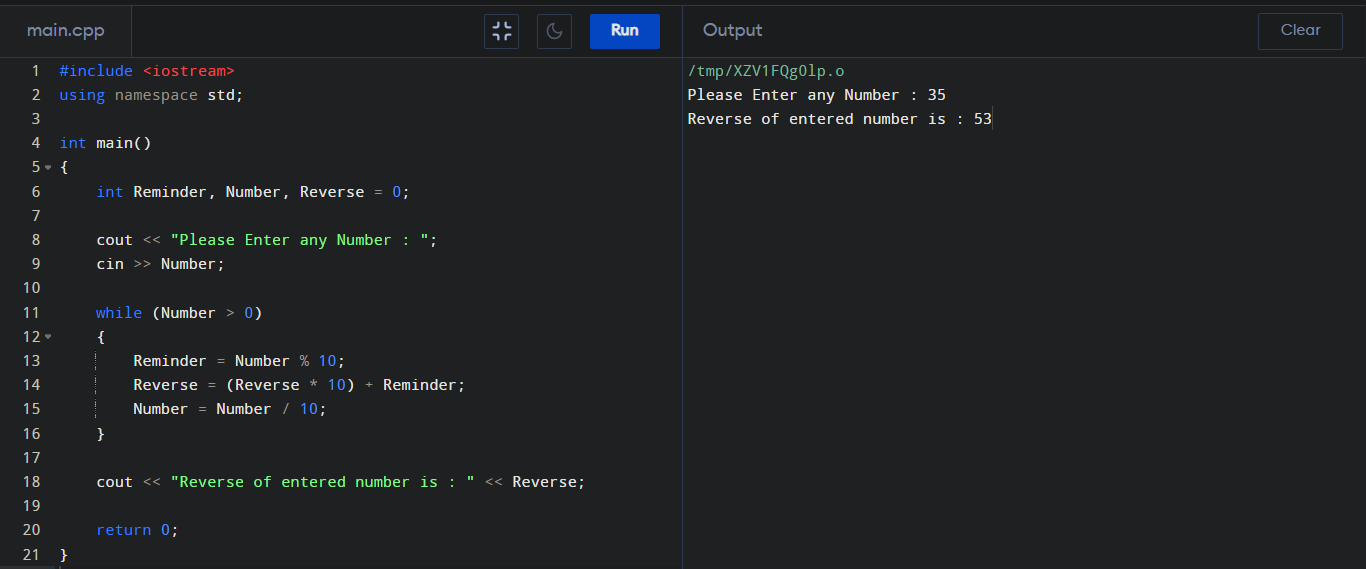## C++ Program to Reverse a Number

How to reverse a number in C++? To reverse a number in C++ program here we can use another variable and also modulus operator.

#### Reverse a Number in C++ :

``````
#include <iostream>
using namespace std;

int main()
{
int Reminder, Number, Reverse = 0;

cout << "Please Enter any Number : ";
cin >> Number;

while (Number > 0)
{
Reminder = Number % 10;
Reverse = (Reverse * 10) + Reminder;
Number = Number / 10;
}

cout << "Reverse of entered number is : " << Reverse;

return 0;
}
``````

#### Output :